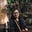Related Tags

java
communitycreator

# How to find common elements in three sorted arraysTahanima Chowdhury

### Problem overview

In this shot, we will learn how to print all the common integers in three arrays sorted in non-decreasing order.

For example, consider the three arrays below:

[1, 2, 3]
[1, 3, 4, 5]
[1, 2, 3, 4, 6]


All the common integers in the arrays are as follows:

1
3


### Solution algorithm

We know beforehand that the arrays are sorted in non-decreasing order. When we find a common integer, we want to move ahead in each of the arrays to look for another common integer. Otherwise, we are certain that the smaller integer among the three integers cannot be common.

This is because at least one of the other integers is a larger integer and we only have larger integers as we move ahead in that array. In this scenario, we want to move ahead in only the array that contains the smaller integer.

#### Steps

• Create three variables that will store the sizes of the arrays. This will help to determine whether all the integers in a given array are traversed through.
• Let the variables be n1, n2, and n3.
• Create and initialize three variables that will point to the indices of the arrays. The starting index is 0.
• Let the variables be i, j, and k.
1. Repeat the following steps until we reach the end of any one of the arrays:
• Check whether the integers appointed by i, j, and k are equal or not.
• If they are equal, print any of the integers and increase i, j, and k by 1.
• Otherwise, increase the index that points to the smaller integer by 1.

### Complexity

• Time Complexity: $O(N)$, where $N$ is the maximum size among the sizes of the arrays. In the worst case we traverse till the array having the maximum size is evaluated.

• Space Complexity: $O(1)$. We only use some variables that occupy constant extra space.

### Code

public class Main {

public static void findCommonElements(int[] arr1, int[] arr2, int[] arr3) {
// stores size of arr1
int n1 = arr1.length;

// stores size of arr2
int n2 = arr2.length;

// stores size of arr3
int n3 = arr3.length;

// stores starting index of arr1
int i = 0;

// stores starting index of arr2
int j = 0;

// stores starting index of arr3
int k = 0;

/*
* traverses the arrays until
* we reach the end of
* one of the arrays
*/
while (i < n1 && j < n2 && k < n3) {
// if the integers appointed by i, j and k are equal
if (arr1[i] == arr2[j] && arr2[j] == arr3[k]) {
// prints the common integer
System.out.println(arr1[i]);

// increases i, j and k by 1
i++; j++; k++;
}

/*
* otherwise, if arr1[i] < arr2[j]
* we have already found one smaller integer
* which is arr1[i]
*/
else if (arr1[i] < arr2[j]) {
i++; // increases i by 1
}

/*
* otherwise, if arr2[j] < arr3[k]
* we have got a smaller integer
* which is arr2[j]
*/
else if (arr2[j] < arr3[k]) {
j++; // increases j by 1
}

/*
* otherwise, we consider arr3[k] to be smaller
*/
else {
k++; // increases k by 1
}
}
}

public static void main(String[] args) {
int arr1[] = {1, 2, 3};
int arr2[] = {1, 3, 4, 5};
int arr3[] = {1, 2, 3, 4, 6};

findCommonElements(arr1, arr2, arr3);
}
}

RELATED TAGS

java
communitycreator

CONTRIBUTORTahanima Chowdhury
RELATED COURSES

View all Courses

Keep Exploring

Learn in-demand tech skills in half the time